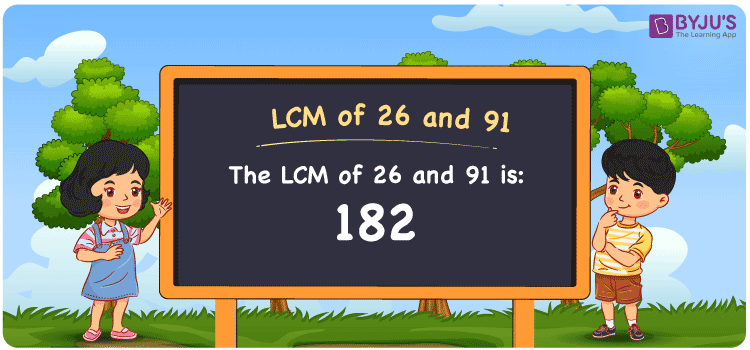Checkout JEE MAINS 2022 Question Paper Analysis : Checkout JEE MAINS 2022 Question Paper Analysis :

# LCM of 26 and 91

LCM of 26 and 91 is 182. The Lowest Common Multiple (LCM) of any two or more natural numbers is the number that is the lowest of their common multiples. Students can go through the Least Common Multiple (LCM) to understand the concept in a better way. We can use prime factorisation, listing multiples and the division method to find the LCM of given numbers. Learn the simple tricks of finding the least common multiple of 26 and 91 in a precise manner in this article.

## What is LCM of 26 and 91?

The answer to this question is 182.## How to Find LCM of 26 and 91?

The methods used to find the LCM of 26 and 91 are given below:

• Prime Factorisation
• Division method
• Listing the multiples

### LCM of 26 and 91 Using Prime Factorisation Method

By prime factorisation method, we can write 26 and 91 as the product of prime numbers, such that;

26 = 2 × 13

91 = 7 × 13

LCM (26, 91) = 2 × 7 × 13 = 182

### LCM of 26 and 91 Using Division Method

In this method, we divide the numbers 26 and 91 by their prime factors to determine their LCM. The product of these divisors denotes the least common multiple of 26 and 91. This can be represented as below.

 2 26 91 7 13 91 13 13 13 x 1 1

No more further division can be done.

Hence, LCM (26, 91) = 2 × 7 × 13 = 182

### LCM of 26 and 91 Using Listing the Multiples

By listing the multiples of 26 and 91, we can determine the least common multiple of 26 and 91 in an efficient way. The below table shows the multiples of 26 and 91.

 Multiples of 26 Multiples of 91 26 91 52 182 78 273 104 364 130 455 156 546 182 637 208 728 234 819

LCM (26, 91) = 182

## Related Articles

LCM Calculator

HCF and LCM

LCM of Two Numbers

Prime Factorization and Division Method for LCM and HCF

LCM Formula

LCM with Examples

## Video Lesson on Applications of LCM## Solved Examples

Q. 1: Calculate the LCM if the product of two numbers is 2366 and their GCD is 13?

Solution: Given

GCD = 13

Product of numbers = 2366

We know that,

LCM × GCD = Product of numbers

LCM = Product of numbers/GCD

LCM = 2366/13

LCM = 182

Q. 2: Find the LCM of 26 and 91 using prime factorisation method.

Solution: 26 = 2 × 13

91 = 7 × 13

LCM (26, 91) = 2 × 7 × 13 = 182

Hence the LCM of 26 and 91 is 182.

## Frequently Asked Questions on LCM of 26 and 91

### What is the LCM of 26 and 91?

The LCM of 26 and 91 is 182.

### List out the methods used to find the LCM of 26 and 91.

The LCM of 26 and 91 can be determined by the following methods

Prime factorisation

Division Method

Listing the multiples

### How do you find the LCM of 26 and 91 by using the method of listing the multiples?

First list the multiples of 26 and 91

26 = 26, 52, 78, 104, 130, 156, 182,…..

91 = 91, 182, 273, 364,455, 546,…..

The LCM is 182

Therefore the LCM of 26 and 91 is 182.